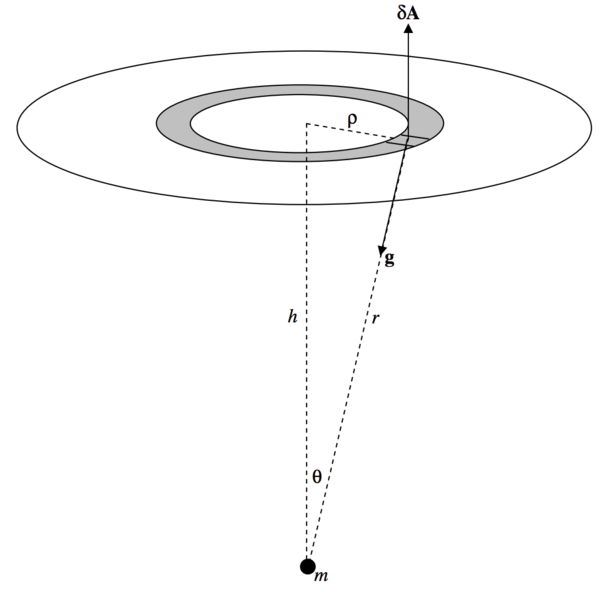$$\require{cancel}$$

# 5.6: Calculating Surface Integrals

•• Contributed by Jeremy Tatum
• Emeritus Professor (Physics & Astronomy) at University of Victoria

While the concept of a surface integral sounds easy enough, how do we actually calculate one in practice? In this section I do two examples.

Example $$\PageIndex{1}$$

In Figure $$\text{V.19}$$ I show a small mass $$m$$, and I have surrounded it with a cylinder or radius $$a$$ and height $$2h$$. The problem is to calculate the surface integral $$∫ \textbf{g} \cdot d\textbf{A}$$ through the entire surface of the cylinder. Of course we already know, from Gauss’s theorem, that the answer is $$= −4 \pi Gm$$, but we would like to see a surface integral actually carried out.$$\text{FIGURE V.19}$$

I have drawn a small element of the surface. Its area $$δA$$ is $$dz$$ times $$aδ \phi$$, where $$\phi$$ is the usual azimuthal angle of cylindrical coordinates. That is, $$δA = a \ δz \ δ\phi$$. The magnitude $$g$$ of the field there is $$Gm/r^2$$ , and the angle between $$\textbf{g}$$ and $$d\textbf{A}$$ is $$90^\circ + θ$$. The outward flux through the small element is

$\textbf{g} \cdot δ \textbf{A} = \frac{Gma \cos (θ+90^\circ) δz δ \phi}{r^2}.$

(This is negative – i.e. it is actually an inward flux – because $$\cos (θ + 90^\circ ) = −\sin θ$$.) When integrated around the elemental strip $$δz$$, this is $$- \frac{2 \pi Gma \sin θ δz}{r^2}.$$ To find the flux over the total curved surface, let’s integrate this from $$z = 0$$ to $$h$$ and double it, or, easier, from $$θ = \pi/2$$ to $$α$$ and double it, where $$\tan α = a/h$$. We’ll need to express $$z$$ and $$r$$ in terms of $$θ$$ (that’s easy:- $$z = a \cot θ$$ and $$r = a \csc θ$$),and the integral becomes

$4 \pi Gm \int_{\pi/2}^α \sin θ \ d θ = -4 \pi Gm \cos α \label{5.6.1} \tag{5.6.1}$

Let us now find the flux through one of the flat ends of the cylinder.$$\text{FIGURE V.20}$$

This time, $$δA = ρ \ δρ \ δ\phi$$, $$g = Gm/r^2$$ and the angle between $$\textbf{g}$$ and $$δ\textbf{A}$$ is $$180^\circ − θ$$. The outwards flux through the small element is $$\frac{Gmρ \cos (180^\circ - θ)δρδ\phi}{r^2}$$ and when integrated around the annulus this becomes $$-\frac{2\pi Gm \cos θ \ ρδρ}{r^2}$$. We now have to integrate this from $$ρ = 0$$ to $$a$$, or, better, from $$θ = 0$$ to $$α$$. We have $$r = h \sec θ$$ and $$ρ = h \tan θ$$, and the integral becomes

$-2 \pi Gm \int_0^α \sin θ d θ = - 2\pi Gm (1- \cos α). \label{5.6.2} \tag{5.6.2}$

There are two ends, so the total flux through the entire cylinder is twice this plus equation $$\ref{5.6.1}$$ to give

$\phi = -4\pi Gm, \label{5.6.3} \tag{5.6.3}$

as expected from Gauss’s theorem.

Example $$\PageIndex{2}$$

In figure $$\text{V.21}$$ I have drawn (part of) an infinite rod whose mass per unit length is $$λ$$. I have drawn around it a sphere of radius $$a$$. The problem will be to determine the total normal flux through the sphere. From Gauss’s theorem, we know that the answer must be $$−8πGαλ$$.$$\text{FIGURE V.21}$$

The vector $$δ\textbf{A}$$ representing the element of area is directed away from the centre of the sphere, and the vector $$\textbf{g}$$ is directed towards the nearest point of the rod. The angle between them is $$θ + 90^\circ$$. The magnitude of $$δ\textbf{A}$$ in spherical coordinates is $$a^2 \sin θδθδ \phi$$, and the magnitude of $$\textbf{g}$$ is (see equation 5.4.15) $$\frac{2Gλ}{a \sin θ}.$$ The dot product $$\textbf{g}⋅δ \textbf{A}$$ is

$\frac{2Gλ}{a \sin θ} \cdot a^2 \sin θδθδ \phi \cdot \cos ( θ + 90^\circ) = - 2 G λ a \sin θδθδ \phi . \label{5.6.4} \tag{5.6.4}$

To find the total flux, this must be integrated from $$\phi = 0$$ to $$2 \pi$$ and from $$θ = 0$$ to $$\pi$$. The result, as expected, is $$−8 \pi Gαλ$$.